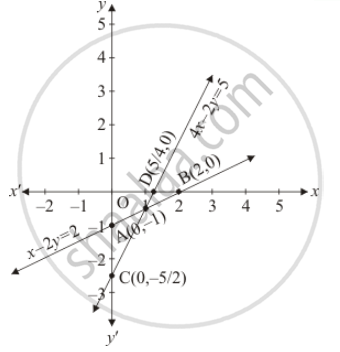Share

# Determine, Graphically Whether the System of Equations X − 2y = 2, 4x − 2y = 5 is Consistent Or In-consistent. - CBSE Class 10 - Mathematics

ConceptGraphical Method of Solution of a Pair of Linear Equations

#### Question

Determine, graphically whether the system of equations x − 2y = 2, 4x − 2y = 5 is consistent or in-consistent.

#### Solution

The given equations are

x - 2y = 2 ....(i)

4x - 2y = 5  ....(ii)

Putting x = 0 in equation (i) we get

=> 0 - 2y = 2

=> y = -1

=> x = 0, y = -1

Putting y = 0 in equation (i) we get

=> x - 2 xx 0 = 2

=> x = 2

=> x = 2, y = 0

Use the following table to draw the graph.

 x 0 2 y -1 0

Draw the graph by plotting the two points A(0,-1), B(2,0) from table.4x - 2y = 5 .....(ii)

Putting x = 0 in equation (ii) we get

=> 4 xx 0 - 2y = 5

=> y = -5/2

=> x = 0, y = -5/2

Putting y = 0in equation (ii) we get

=> 4x - 2 xx 0 = 5

=> y = 5/4

=> x = 5/4, y = 0

Use the following table to draw the graph

 x 0 5/4 y -5/2 0

Draw the graph by plotting the two points C(0, -5/2), D(5/4,0) from the table.

It has a unique solution.

Hence the system of equations is consistent

Is there an error in this question or solution?

#### APPEARS IN

Solution Determine, Graphically Whether the System of Equations X − 2y = 2, 4x − 2y = 5 is Consistent Or In-consistent. Concept: Graphical Method of Solution of a Pair of Linear Equations.
S